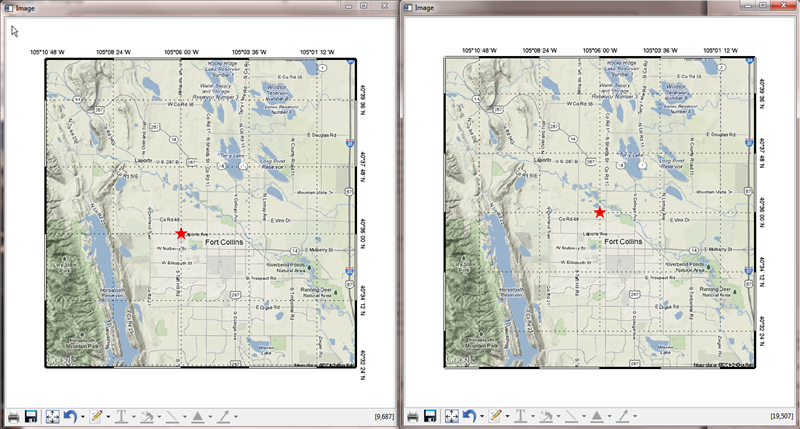# Map Function LIMIT Keyword is BrokenQUESTION: I'm getting really screwy results when I use the LIMIT keyword with my map projections using the Map function in IDL 8.2. Is this keyword broken?ANSWER: Yes, the LIMIT keyword to the Map function is broken in IDL 8.2. And, even worse, you can get different results depending on when and where you use it.

The LIMIT keyword is used most often when you are trying to associate a map projection space with an image that has been gridded using a specific map projection. GeoTiff files are good examples of this. If you try to set up the map projection space by setting keywords in the IDL 8.2 Image function, and you use the LIMIT keyword to help set up that space, the result will be a completely blank graphics window!

```   img = Image(geoTiffImage, Map_Projection='Mercator', Ellipsoid='WGS 84', \$
LIMIT=mapLimits, ... )
```

If you separate the image display from the map projection set-up, then the LIMIT keyword appears to work, but returns erroneous results.

```   img = Image(geoTiffImage)
mp  = Map('Mercator', Ellipsoid='WGS 84', LIMIT=mapLimits, /Current, ... )
```

For example, in a previous article in which I attempted to navigate and display box axes on an image, I had to set the LIMIT and BOX_AXES keywords after the fact, like this.

```   obj = Image(googleImage, MAP_PROJECTION='mercator', \$
ELLIPSOID='WGS 84', GRID_UNITS=1, \$
XRANGE=xrange, YRANGE=yrange, \$
DIMENSIONS=[700,700], POSITION=[0.1, 0.1, 0.9, 0.9], \$
IMAGE_DIMENSIONS=[xdim,ydim], IMAGE_LOCATION=[xloc,yloc])
sym = Symbol(centerLon, centerLat, 'star', /DATA, SYM_COLOR='red', SYM_SIZE=3, SYM_FILLED=1)
obj.mapprojection.mapgrid.LINESTYLE = 1
obj.mapprojection.mapgrid.GRID_LONGITUDE = 0.04
obj.mapprojection.mapgrid.GRID_LATITUDE = 0.03
obj.mapprojection.mapgrid.LABEL_POSITION = 1
obj.mapprojection.mapgrid.LONGITUDE_MIN=-105.18
obj.mapprojection.mapgrid.LATITUDE_MIN=40.54
obj.mapprojection.mapgrid.BOX_AXES = 1
obj.mapprojection.LIMIT = limit
```

When I tried separating the image display and the map projection, but otherwise using exactly the same input parameters, I got erroneous results. The code I used was this.

```   imgObj = Image(googleImage, POSITION=[0.1, 0.1, 0.9, 0.9], DIMENSIONS=[700,700])
mp = Map('mercator', ELLIPSOID='WGS 84', CENTER_LONGITUDE=centerLon, \$
LIMIT=limit, /BOX_AXES, POSITION=[0.1, 0.1, 0.9, 0.9], /CURRENT, \$
GRID_LONGITUDE=0.04, GRID_LATITUDE=0.03, LABEL_POSITION=1, \$
LONGITUDE_MIN=-105.18, LATITUDE_MIN=40.54, LINESTYLE=1)
sym = Symbol(centerLon, centerLat, 'star', /DATA, SYM_COLOR='red', SYM_SIZE=3, SYM_FILLED=1)
```

You see the incorrect results on the left in the figure below (click the image to expand the results). The correct results were obtained by setting the LIMIT values after I create the map projection object. You see the correct results on the right in the figure below.

```   imgObj = Image(googleImage, POSITION=[0.1, 0.1, 0.9, 0.9], DIMENSIONS=[700,700])
mp = Map('mercator', ELLIPSOID='WGS 84', CENTER_LONGITUDE=centerLon, \$
LIMIT=limit, /BOX_AXES, POSITION=[0.1, 0.1, 0.9, 0.9], /CURRENT, \$
GRID_LONGITUDE=0.04, GRID_LATITUDE=0.03, LABEL_POSITION=1, \$
LONGITUDE_MIN=-105.18, LATITUDE_MIN=40.54, LINESTYLE=1)
mp.LIMIT = limit
sym = Symbol(centerLon, centerLat, 'star', /DATA, SYM_COLOR='red', SYM_SIZE=3, SYM_FILLED=1)
```Using the LIMIT keyword can result in incorrect results. Always set the LIMIT after creating the map projection object.